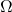# The effect of omega invariant on some topological graph indices

### Full PDFcreative_2021_30_2_175_180

Issue no:

For a realizable set of non-negative integers, it is well-known that there are many ways of realizing it as a graph having this set as degree sequence. For a given degree sequence, a new graph invariant denoted bywhich is related to the cyclomatic number and Euler characteristic is recently defined. It is already shown that this new invariant releases important combinatorial properties and gives direct information compared to the better known Euler characteristic on many normal or extremal problems related to the realizability, cyclicness, components, chords, loops, connectedness, etc. Many similar classification problems can be solved by means of. Topological graph indices are used in applications of graph theory as they give us some mathematical results by means of some graph model of a real life situation which can frequently be used in other applied sciences. Therefore it is one of the main problems of graph theory to search for the possible values of these indices. Many problems dealing with the range of a topological index become easier if we could determine the lower and upper bounds for this topological index. In this paper, we study the change of several topological graph indices, the first and second Zagreb indices, forgotten index, sigma index and Narumi-Katayama index amongst all possible (fundamental).